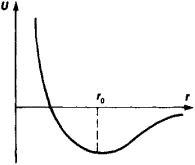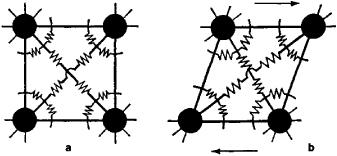# elasticity

(redirected from blood vessel elasticity)
Also found in: Dictionary, Thesaurus, Medical, Financial.

## elasticity,

the ability of a body to resist a distorting influence or stress and to return to its original size and shape when the stress is removed. All solids are elastic for small enough deformations or strains, but if the stress exceeds a certain amount known as the elastic limit, a permanent deformation is produced. Both the resistance to stress and the elastic limit depend on the composition of the solid. Some different kinds of stresses are tension, compression, torsion, and shearing (see strength of materialsstrength of materials,
measurement in engineering of the capacity of metal, wood, concrete, and other materials to withstand stress and strain. Stress is the internal force exerted by one part of an elastic body upon the adjoining part, and strain is the deformation or change in
). For each kind of stress and the corresponding strain there is a modulus, i.e., the ratio of the stress to the strain; the ratio of tensile stress to strain for a given material is called its Young's modulusYoung's modulus
[for Thomas Young], number representing (in pounds per square inch or dynes per square centimeter) the ratio of stress to strain for a wire or bar of a given substance.
. Hooke's law [for Robert HookeHooke, Robert
, 1635–1703, English physicist, mathematician, and inventor. He became curator of experiments for the Royal Society (1662), professor of geometry at Gresham College (1665), and city surveyor of London after the great 1666 fire.
] states that, within the elastic limit, strain is proportional to stress.

## Elasticity

The ability of a material to instantly deform under load and to recover its original shape when the load is removed.
The following article is from The Great Soviet Encyclopedia (1979). It might be outdated or ideologically biased.

## Elasticity

the property whereby macroscopic bodies resist a change in their size or shape under the action of mechanical stresses. When the applied stress is removed, an elastically deformed body recovers its original size and shape.

The elasticity of a body is due to the forces by which the atoms composing the body interact. In solids, at a temperature of absolute zero and in the absence of externally applied stresses, the atoms occupy equilibrium positions at which the sum of all forces acting on each atom from the direction of the other atoms is equal to zero and the potential energy of the atom is minimal. In addition to the forces of attraction and repulsion, which depend solely on the interatomic distance (Figure 1), angular forces, which depend on the bond angles between the lines that connect a given atom to its various neighbors (Figure 2), also act in polyatomic molecules and macroscopic bodies. At the equilibrium values of the bond angles, the angular forces are also equalized. The energy of a macroscopic body depends on the interatomic distances and bond angles and takes a minimum value at the equilibrium values of these parameters.Figure 1. Plot of the potential interaction energy of two atoms against interatomic distance r. The equilibrium state r0 corresponds to the smallest value of the potential energy. At this distance, the interatomic forces of attraction and repulsion are equalized.

Under the action of externally applied stresses, the atoms are displaced from their equilibrium positions. This process is accompanied by an increase in the potential energy of the body by an amount equal to the work of the externally applied stresses in changing the size and shape of the body. After the externally applied stresses are removed, the configuration of an elastically deformed body with nonequilibrium interatomic distances and bond angles turns out to be unstable and spontaneously reverts to the equilibrium state; more accurately, the atoms oscillate about their equilibrium positions. The excess potential energy accumulated in the body is transformed into the kinetic energy of the vibrating atoms, that is, into heat. As long as the deviations of the interatomic distances and bond angles from their equilibrium values are small, such deviations are proportional to the forces acting between the atoms, just as the extension or compression of a spring is proportional to the applied force. Therefore, a body may be represented as a set of spherical atoms connected by springs whose orientations are fixed by other springs (Figure 2). The elastic constants of the springs determine the moduli of elasticity of the material. The elastic deformation of the body is proportional to the applied stress; that is, it is given by Hooke’s law, which is the basis of the theory of elasticity and the strength of materials.Figure 2. Sphere model of a cubic crystal lattice: (a) in equilibrium, (b) under the action of an externally applied shearing stress

At finite temperatures below the melting point, small thermal vibrations of the atoms about the equilibrium positions occur even when no external stress is applied or removed. Consequently, the moduli of elasticity of a material depend on temperature, but the essence of the phenomena considered is not changed.

In a liquid, the thermal vibrations have an amplitude comparable to the equilibrium distance r0; as a result, the atoms easily move about relative to one another, and they do not resist shearing stresses if such stresses are applied at a rate substantially lower than the rate of the thermal vibrations. Therefore, liquids, like gases, are not elastic with respect to shape.

In the gaseous state, the mean interatomic or intermolecular distances are much greater than in the condensed state. The elasticity of gases or vapors is determined by the thermal motion of the molecules colliding with the walls of the vessel that confines the gas or vapor.

### REFERENCES

Feynman, R., R. Layton, and M. Sands. Feinmanovskie lektsii po fizike, [fasc] 7. Moscow, 1966. Chapters 38 and 39.
Smirnov, A. A. Molekuliarno-kineticheskaia teoriia metallov. Moscow, 1966. Chapter 2.
Frenkel’, Ia. I. Vvedenie v teoriiu metallov, 4th ed. Leningrad, 1972. Chapter 2.

A. N. ORLOV

## elasticity

[i‚las′tis·əd·ē]
(mechanics)
The property whereby a solid material changes its shape and size under action of opposing forces, but recovers its original configuration when the forces are removed.
The existence of forces which tend to restore to its original position any part of a medium (solid or fluid) which has been displaced.
McGraw-Hill Dictionary of Scientific & Technical Terms, 6E, Copyright © 2003 by The McGraw-Hill Companies, Inc.

## Elasticity

The property whereby a solid material changes its shape and size under the action of opposing forces, but recovers its original configuration when the forces are removed. The theory of elasticity deals with the relations between the forces acting on a body and the resulting changes in configuration, and is important in many branches of science and technology, for instance, in the design of structures, in the theory of vibration and sound, and in the study of the forces between atoms in crystal lattices.

The forces acting on a body are expressed as stresses and measured as force per unit area. Thus if a bar ABCD of square cross section (illus. a) is fixed at one end and subjected to a force F uniformly distributed over the other end DC, the stress is F/(DC)2. This stress causes the bar to become longer and thinner and to assume the shape A′B′C′D′. The strain is measured by the ratio (change in length)/(original length), that is, by (B′C′ - BC)/(BC). According to Hooke's law, stress is proportional to strain, and the ratio of stress to strain is therefore a constant, in this case the Young's modulus, denoted by E, so that E = F(BC)/(DC)2 (B′C′ - BC). See Stress and strain, Young's modulus

Poisson's ratio σ is the ratio of lateral strain to longitudinal strain so that σ = BC(DC - D′C′)/DC(B′C′ - BC). The bar of illustration a is in a state of tension, and the stress is tensile; if the force F were reversed in direction, the stress would be compressive. Stresses of this type are called direct or normal stresses; a second type of stress, known as tangential or shear stress, is shown in illus. b. In this case, the configuration ABCD becomes ABC′D′, with the shear forces F acting in the directions AB and CD. The shear strain is measured by the angle Θ, and if the body is originally a cube, the shear stress is F/(DC). The ratio of stress to strain, F/(DC)2 Θ, is the shear or rigidity modulus G, which measures the resistance of the material to change in shape without change in volume.

A further elastic constant, the bulk modulus k, measures the resistance to change in volume without changes in shape, and is shown in illus. c. The original configuration is represented by the circle AB, and under a hydrostatic (uniform) pressure P, the circle AB becomes the circle A′B′. The bulk modulus is then k = Pvv, where Δv/v is the volumetric strain. The reciprocal of the bulk modulus is the compressibility.

The elastic constants may be determined directly in the way suggested by their definitions; for instance, Young's modulus can be determined by measuring the relative extension of a rod or wire subjected to a known tensile stress. Less direct methods are, however, usually more convenient and accurate. Prominent among these are the dynamic methods involving frequency of vibration and velocity of sound propagation. The elastic constants can be expressed in terms of frequency of (or velocity in) regularly shaped specimens, together with the dimensions and density, and by measuring these quantities, the elastic constants can be found. The elastic constants can also be determined from the flexure and torsion of bars.

In practice, stress is only proportional to strain, and the strain is only completely recoverable within certain limits called the elastic limits of the material. Above the elastic limits, the material is subject to time-dependent effects, and as the stress is further increased, the ultimate strength of the material is approached.

McGraw-Hill Concise Encyclopedia of Engineering. © 2002 by The McGraw-Hill Companies, Inc.

## elasticity

The property of a body that causes it to tend to return to its original shape after deformation (as stretching, compression, or torsion).
McGraw-Hill Dictionary of Architecture and Construction. Copyright © 2003 by McGraw-Hill Companies, Inc.

## elasticity

The ability of a material to be elongated, or stretched, by pulling on it and to return to its original size and shape after the pulling stops.